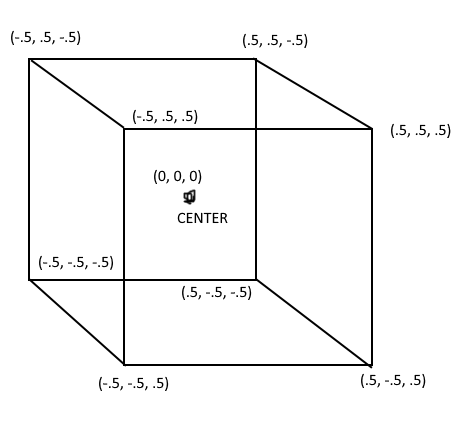# Help with random part generator in region

I want a random part generator, but instead of hard coded XYZ placements, I want the parts to spawn inside of a part. I found this script that kind of does the job:

``````while wait(2.5) do

local Part = script.Parent

local Speed = game.ServerStorage.Speed_Orb:Clone()

Speed.CFrame = Part.CFrame * CFrame.new(math.random(-Part.Size.X/2, Part.Size.X/2),math.random(-Part.Size.Y/2, Part.Size.Y/2),math.random(-Part.Size.X/2, Part.Size.X/2))
Speed.Parent = workspace
wait(2.5)
Speed:Destroy()
end
``````

(I modified it to do what I need by the way.)

but sometimes, the part spawns outside of the region, it actually happens a lot. Is there a better alternative to this method? Or is it just a small flaw.

Try using the image below from this post to find the vertices.I don’t really understand what any of that means? Is there a way that you can explain it a bit, I don’t really understand much geometry.

Your problem is that you use the part’s X size value instead of the Z size value when moving the speed orb. It’s supposed to be:

``````Speed.CFrame = Part.CFrame * CFrame.new(math.random(-Part.Size.X/2, Part.Size.X/2),math.random(-Part.Size.Y/2, Part.Size.Y/2),math.random(-Part.Size.Z/2, Part.Size.Z/2))
``````
2 Likes

A little different from the way you were doing it, but it does keep all the parts inside the region. Here is the code. You’ll need to modify it to fit your needs.

``````local function CreatePart()
local NewPart = Instance.new('Part', workspace);
NewPart.Anchored = true;
NewPart.BrickColor = BrickColor.Random();
NewPart.Size = Vector3.new(1,1,1);
NewPart.Material = Enum.Material.SmoothPlastic;

return NewPart
end

local function CreateRandomPosition(Part0, Part1Size)
local PartPos = Part0.Position;
local PartSize = Part0.Size;
local r = math.random;

local Boundry__x0 = (PartPos.X - PartSize.X/2) + Part1Size.X/2;
local Boundry__x1 = (PartPos.X + PartSize.X/2) - Part1Size.X/2;

if Boundry__x1 < Boundry__x0 then
Boundry__x1 = Boundry__x0 + 0.05
end

local Boundry__y0 = (PartPos.Y - PartSize.Y/2) + Part1Size.Y/2;
local Boundry__y1 = (PartPos.Y + PartSize.Y/2) - Part1Size.Y/2;

if Boundry__y1 < Boundry__y0 then
Boundry__y1 = Boundry__y0 + 0.05
end

local Boundry__z0 = (PartPos.Z - PartSize.Z/2) + Part1Size.Z/2;
local Boundry__z1 = (PartPos.Z + PartSize.Z/2) - Part1Size.Z/2;

if Boundry__z1 < Boundry__z0 then
Boundry__z1 = Boundry__z0 + 0.05
end

local RndPos = Vector3.new(
r(Boundry__x0 * 100, Boundry__x1 * 100) / 100,
r(Boundry__y0 * 100, Boundry__y1 * 100) / 100,
r(Boundry__z0 * 100, Boundry__z1 * 100) / 100
)

return RndPos
end

while wait() do
pcall(function()
local Prt = CreatePart();
local Pos = CreateRandomPosition(workspace.Part, Prt.Size);

Prt.Position = Pos
end)
end
``````
1 Like

I will try this code soon, looks a little more complex than what my original code was, but sure, lets try this.

1 Like

Alright! I will say, I did run into a bug while making this, and error will throw if the x,y,or z sizes are less than the size of the brick. Let me know if this is an issue and i’ll find a fix.

1 Like

Yeah I noticed that, I was going to edit it and share it, but I just didn’t.

1 Like

I fixed the issue. The parts will not stay completely inside the region because of the size but, it does not throw an error.

1 Like